# Torque Balance

According to Equations (3.185) and (3.186),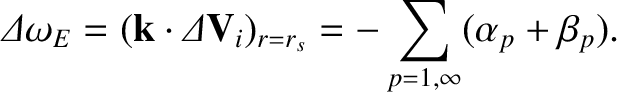(7.25)

In other words, the shift in the E-cross-B frequency at the rational surface,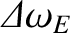, that develops in response to the electromagnetic torque exerted at the surface by the error-field (see Section 3.13), is mirrored by an equal shift in the ion fluid rotation frequency (as well as in the electron fluid rotation frequency). This is the essence of the no-slip constraint, (3.185), which follows from Equations (2.321) and (2.322) because the torque modifies the E-cross-B velocity at the rational surface, but does not affect the diamagnetic velocities.

Equations  (3.190) and (3.191) yield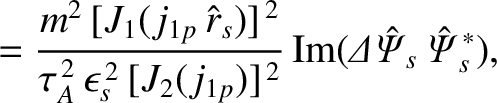(7.26)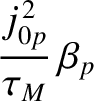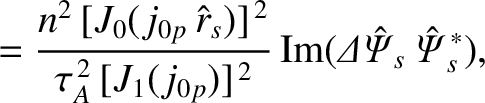(7.27)

where we have set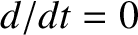(because the error-field is static). Here,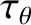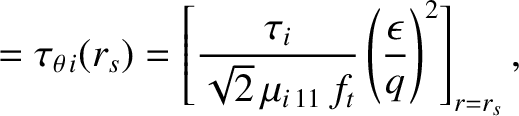(7.28)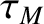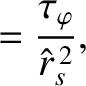(7.29)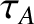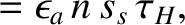(7.30)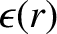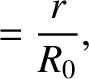(7.31)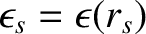,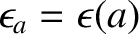, and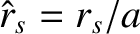. Furthermore, the dimensionless neoclassical viscosity parameter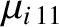is defined in Equation (2.204),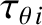is the ion poloidal flow-damping timescale,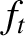the fraction of trapped particles [see Equation (2.202)],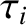the ion-ion collision time [see Equation (2.21)],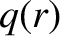the safety-factor profile (see Section 3.2), and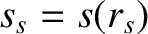the magnetic shear at the rational surface [see Equation (5.28)]. Moreover,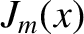denotes a Bessel function, whereas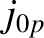and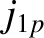denote the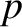th zeros of the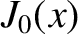and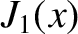Bessel functions, respectively.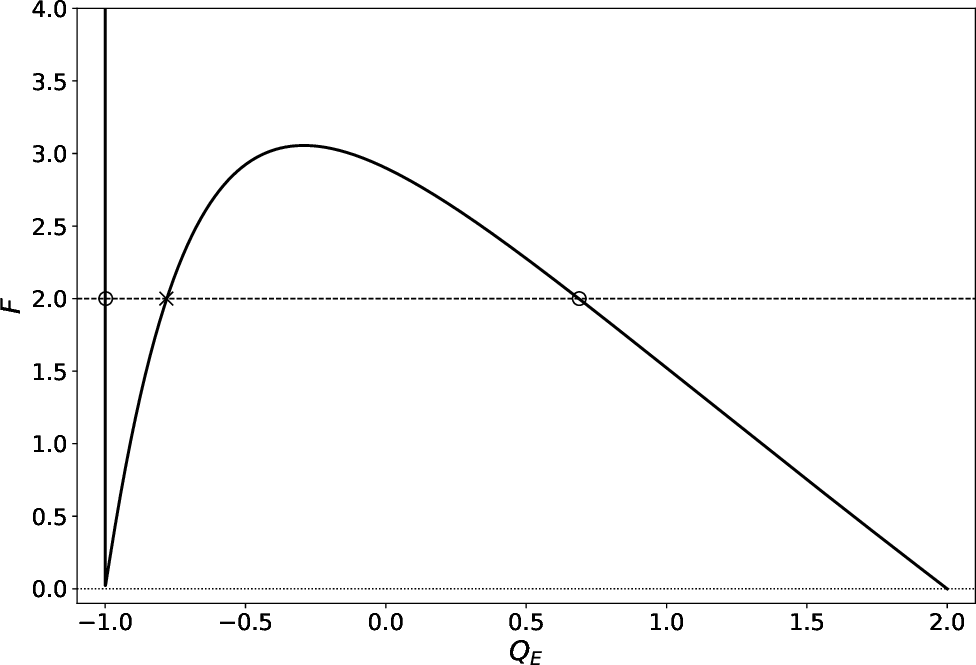Let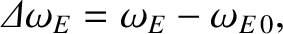(7.32)

where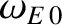is the E-cross-B frequency at the rational surface in the absence of the error-field. It is helpful to define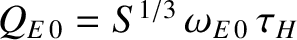. Equations (7.25)–(7.27) can be combined to give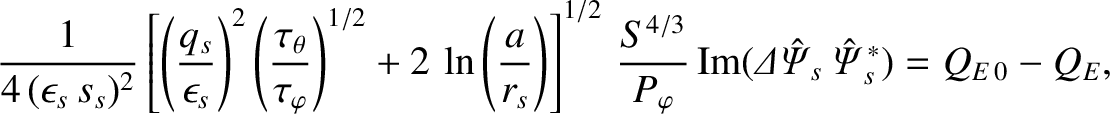(7.33)

where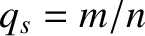. Here, use has been made of the results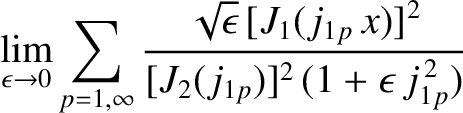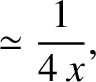(7.34)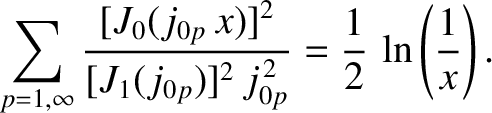(7.35)

Equations (7.9), (7.10), and (7.33) yield the torque balance equation [4,6]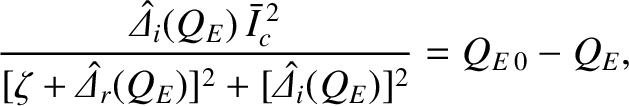(7.36)

where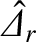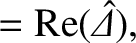(7.37)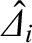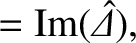(7.38)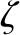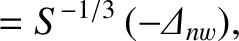(7.39)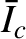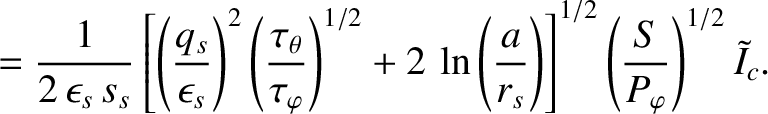(7.40)

The left-hand side of Equation (7.36) represents an electromagnetic braking torque that develops at the rational surface, and acts to halt the local electron fluid rotation. On the other hand, the right-hand side of the equation represents a viscous restoring torque that opposes any changes in the electron fluid rotation at the rational surface.

Equation (7.36) can be rearranged to give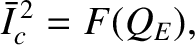(7.41)

where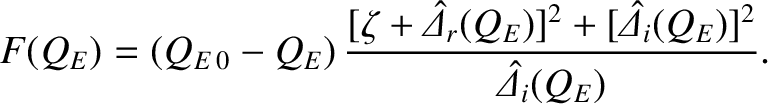(7.42)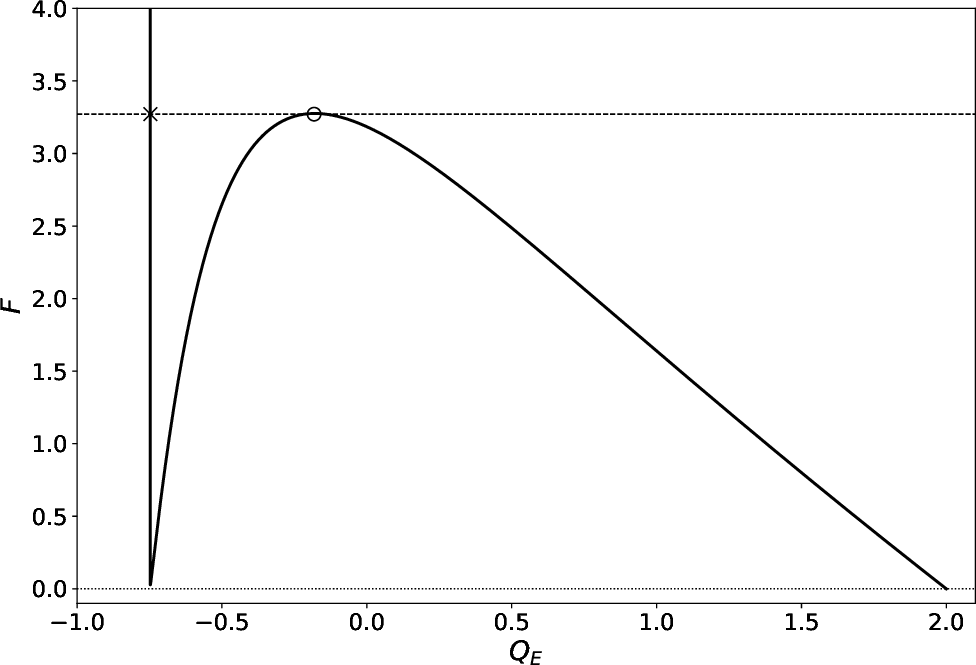Figure 7.3 shows the torque balance function,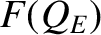, calculated for a low-field tokamak fusion reactor with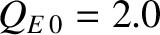. The parameters used in the calculation are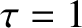,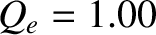,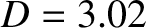,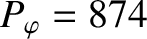, and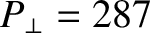, as well as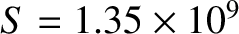,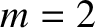, and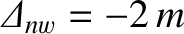. (See Tables 5.1 and 6.1.) Note that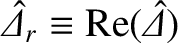andare specified in Equations (7.16) and (7.17), respectively. The parametertakes the value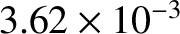. As indicated in the figure, when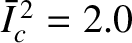there are three possible values of the normalized E-cross-B frequency at the rational surface,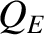, that satisfy Equation (7.41). However, as is easily demonstrated, the middle solution is dynamically unstable . We thus conclude that there are two dynamically stable branches of solutions to the torque balance equation, (7.36). On the high-slip branch (i.e., the rightmost solution in the figure), the electron fluid at the rational surface rotates with respect to the error-field, generating a shielding current that suppresses driven magnetic reconnection. On the low-slip branch (i.e., the leftmost solution in the figure), the electron fluid rotation at the rational surface is arrested, there is no shielding current, and driven magnetic reconnection proceeds unhindered.

Figure 7.4 shows the torque balance function,, calculated for a high-field tokamak fusion reactor with. The parameters used in the calculation are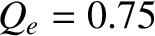, and, as well as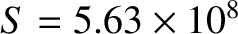,, and. (See Tables 5.1 and 6.1.) Note thatandare specified in Equations (7.22) and (7.23), respectively. The parametertakes the value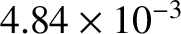. As indicated in the figure, when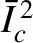attains the critical value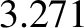, the high-slip and the dynamically unstable solutions of the torque balance equation merge together and annihilate one another. For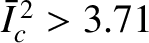, only the low-slip solution branch exists. Thus, if the system is initially on the high-slip solution branch, and the error-field amplitude is raised such thatexceeds the critical value 3.271, then there is a bifurcation from the high-slip to the low-slip solution branch. This bifurcation is associated with the sudden arrest of the electron fluid rotation at the rational surface, the collapse of the shielding current, and the onset of driven magnetic reconnection [4,6]. Of course, the bifurcation corresponds to the error-field penetration phenomenon discussed in Section 7.1.